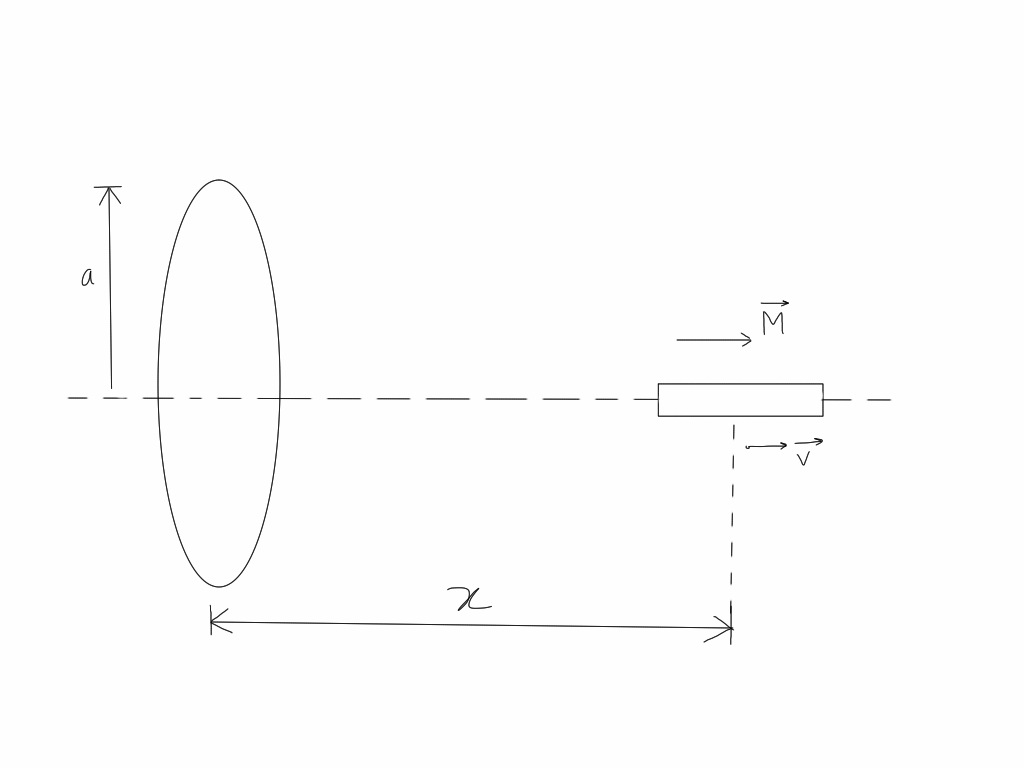# An electricity and magnetism problem by Sudeep SalgiaAlong the axis of a fixed small loop of resistance $R$, a short bar magnet with dipole moment $\vec{M}$ is moved away from it with a velocity $v$. The $\vec{M}$ is oriented such that it points away from the ring as shown in the figure. Given that the radius of the loop is $a$ and distance of the magnet from the center of the loop is $x$, then the magnitude of the force of interaction between the loop and the bar magnet can be writen as $\displaystyle F = \frac{p}{q} . \frac{\mu _0^r M^s a^t v^j}{Rx^k}$ where $j,k,p,q,r,s,t$ are natural numbers with $p$ and $q$ coprime.
Find the value of $j+k+p+q+r+s+t-2$.

Details and Assumptions:

• The bar magnet is short and $a << x$.

• $\mu _0$ is the permeability of vacuum.

• The magnetic field due to the magnet can be considered almost parallel to the axis of the loop.

• Magnitude of force of interaction between two dipoles of moments $M_1$ and $M_2$ is given by $\displaystyle F = \frac{6 \mu_0 M_1 M_2 }{4 \pi x^4}$ where $x$ is the distance between them.

×

Problem Loading...

Note Loading...

Set Loading...